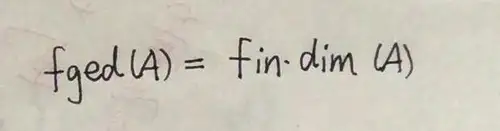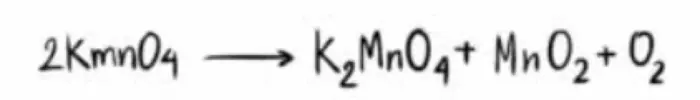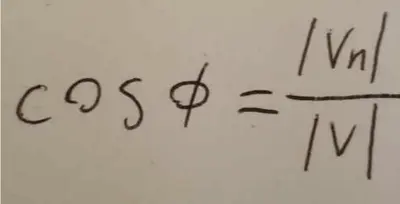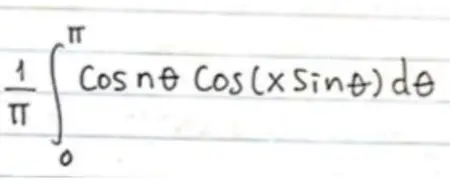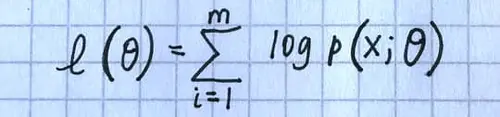# Try Snip on examples of math

Our Snip app allows you to extract math equations from PDFs and books just by taking a screenshot or picture. We convert the images into many useful digital math formats:
• LaTeX (can be used in all LaTeX and Markdown editors),
• MathML (for creating digital and accessible educational materials with math), and
• Asciimath (can be used in web pages)
These images below are examples of the types of equations that you can extract LaTeX, MathML, or Asciimath from. Snip can process all levels of math equations and many different types of writing. For example:
• Printed equations in PDFs (includes scanned PDFs)
• Printed equations in printed textbooks
• Handwritten equations in notebooks
• Handwritten equations on whiteboards
• Handwritten equations on blackboards
• Strokes drawn in our mobile apps’ “Draw” mode

### Examples (printed)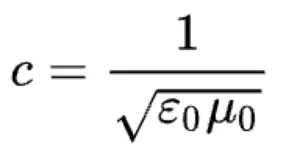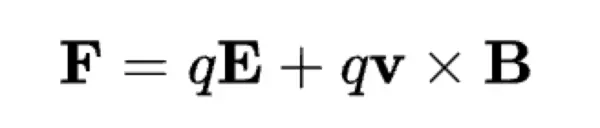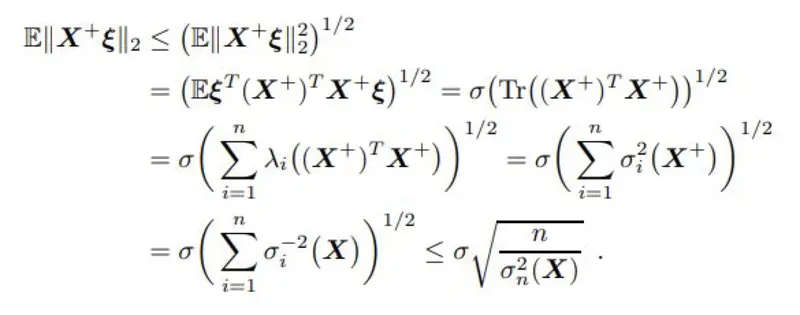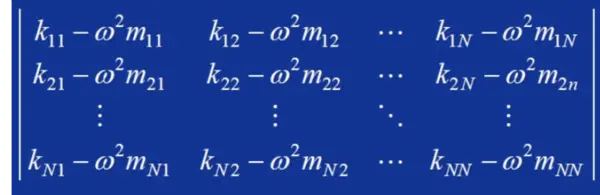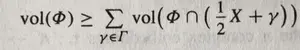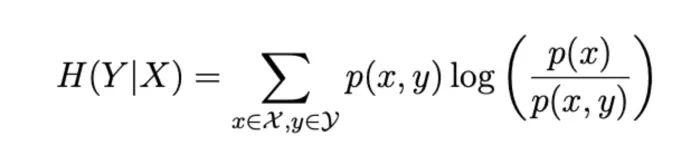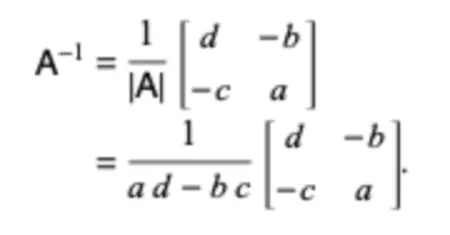### Examples (handwritten)